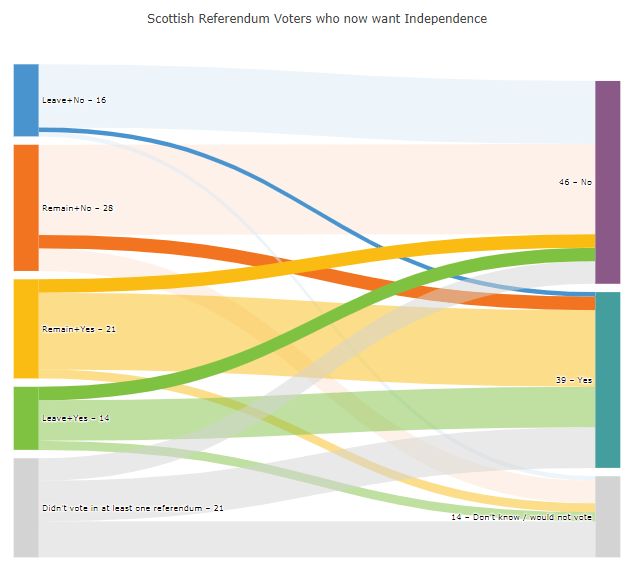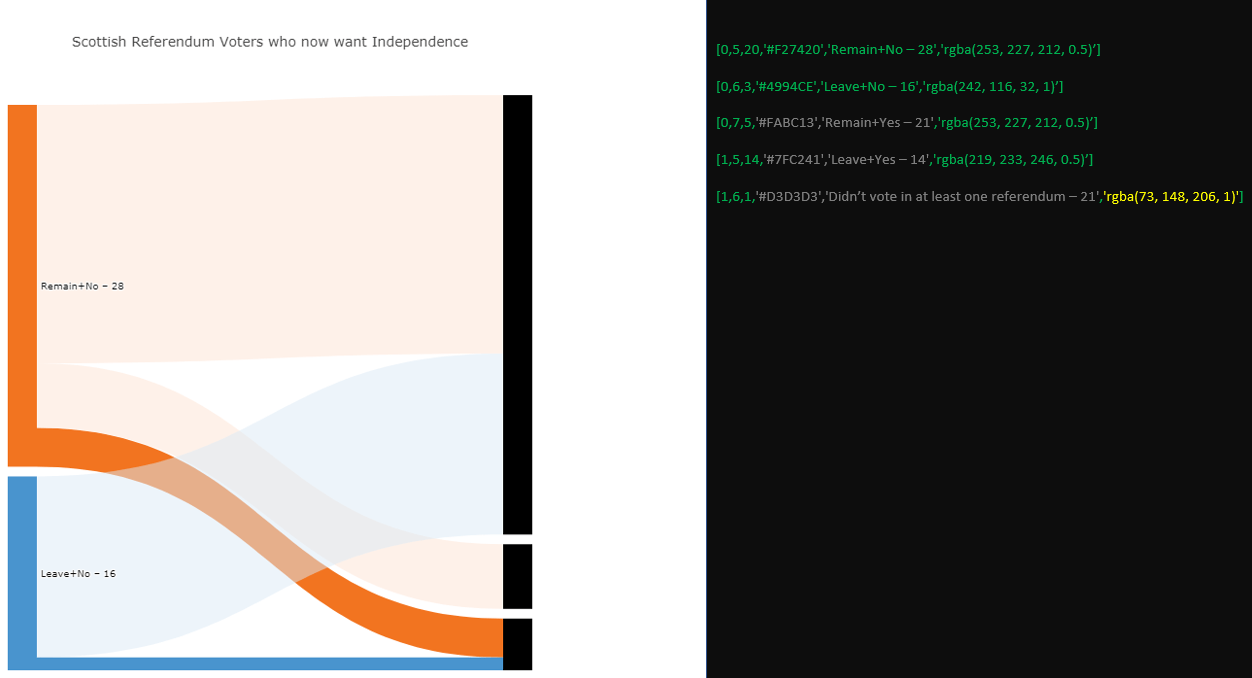# #StackBounty: #python #plotly-python How to define the structure of a sankey diagram using a dataframe?

### Bounty: 50

This may sound like a very broad question, but if you’ll let me describe some details I can assure you it’s very specific. As well as discouraging, frustrating and rage-inducing.

The following plot describes a scottish election and is based on code from plot.ly:

Plot 1:Dataset 1:

``````data = [['Source','Target','Value','Color','Node, Label','Link Color'],
[0,5,20,'#F27420','Remain+No – 28','rgba(253, 227, 212, 0.5)'],
[0,6,3,'#4994CE','Leave+No – 16','rgba(242, 116, 32, 1)'],
[0,7,5,'#FABC13','Remain+Yes – 21','rgba(253, 227, 212, 0.5)'],
[1,5,14,'#7FC241','Leave+Yes – 14','rgba(219, 233, 246, 0.5)'],
[1,6,1,'#D3D3D3','Didn’t vote in at least one referendum – 21','rgba(73, 148, 206, 1)'],
[1,7,1,'#8A5988','46 – No','rgba(219, 233, 246,0.5)'],
[2,5,3,'#449E9E','39 – Yes','rgba(250, 188, 19, 1)'],
[2,6,17,'#D3D3D3','14 – Don’t know / would not vote','rgba(250, 188, 19, 0.5)'],
[2,7,2,'','','rgba(250, 188, 19, 0.5)'],
[3,5,3,'','','rgba(127, 194, 65, 1)'],
[3,6,9,'','','rgba(127, 194, 65, 0.5)'],
[3,7,2,'','','rgba(127, 194, 65, 0.5)'],
[4,5,5,'','','rgba(211, 211, 211, 0.5)'],
[4,6,9,'','','rgba(211, 211, 211, 0.5)'],
[4,7,8,'','','rgba(211, 211, 211, 0.5)']
]
``````

How the plot is built:

I’ve picked up some important details about the behavior of sankey charts from various sources, like:

The challenge:

As you’ll see in the details below, nodes, labels and colors are not applied to the chart in the same order that the source dataframe is structured. Some of that makes perfect sence, since you have various elements that describe the same node like color, targets, values and link color. One node `'Remain+No – 28'` looks like this:And the accompanying part of the dataset looks like this:

``````[0,5,20,'#F27420','Remain+No – 28','rgba(253, 227, 212, 0.5)'],
[0,6,3,'#4994CE','Leave+No – 16','rgba(242, 116, 32, 1)'],
[0,7,5,'#FABC13','Remain+Yes – 21','rgba(253, 227, 212, 0.5)'],
``````

So this part of the source describes a node `` with three corresponding targets `[5, 6, 7]` and three links with the values `[20, 3, 5]`. `'#F27420'` is the orange(ish) color of the node, and the colors `'rgba(253, 227, 212, 0.5)'`, `'rgba(242, 116, 32, 1)'` and `'rgba(253, 227, 212, 0.5)'` describe the colors of the links from the node to some targets. So far, the information that has not been used from the sample above is:

Data sample 2 (partial)

``````[-,-,--'-------','---------------','-------------------'],
[-,-,-,'#4994CE','Leave+No – 16','-------------------'],
[-,-,-,'#FABC13','Remain+Yes – 21','-------------------'],
``````

And that information is used as the remaining elements of the diagram are indtroduced.

So, what’s the question? In the further details below, you’ll see that everything makes sense as long as a new row of data in the dataset inserts a new link, and makes other changes to other elements (colors, labels) if that information has not yet ben used. I’ll be even more specific with the use of two screenshots from a setup I’ve made with plot to the left and code to the right:

The following data sample produces the diagram below following the logic desbribed above:

Data sample 3

``````data = [['Source','Target','Value','Color','Node, Label','Link Color'],
[0,5,20,'#F27420','Remain+No – 28','rgba(253, 227, 212, 0.5)'],
[0,6,3,'#4994CE','Leave+No – 16','rgba(242, 116, 32, 1)'],
[0,7,5,'#FABC13','Remain+Yes – 21','rgba(253, 227, 212, 0.5)'],
[1,5,14,'#7FC241','Leave+Yes – 14','rgba(219, 233, 246, 0.5)'],
[1,6,1,'#D3D3D3','Didn’t vote in at least one referendum – 21','rgba(73, 148, 206, 1)']]
``````

Screenshot 1 – Partial plot with data sample 3THE QUESTION:

Adding the row `[1,7,1,'#8A5988','46 – No','rgba(219, 233, 246,0.5)']` in the dataset produces a new link with a between source `` and target `` but applies color and label to a target 5 at the same time. I would think that the next label to be applied to the chart was `'Remain+Yes – 21'` since it hasn’t been used. But what happens here is that the label `'46 – No'` is applied to Target 5. WHY?

Screenshot 2 – Partial plot with data sample 3 + `[1,7,1,'#8A5988','46 – No','rgba(219, 233, 246,0.5)']` :And how do you discern what is a source and what is a target based on that dataframe?

I know that the question is both strange and hard to answer, but I’m hoping someone has a suggestion. I also know that a dataframe may not be the best source for a sankey chart. Perhaps json instead?

Complete code and data sample for an easy copy&paste for a Jupyter Notebook:

``````import pandas as pd
import numpy as np
import plotly.graph_objs as go
init_notebook_mode(connected=True)

# Original data
[0,5,20,'#F27420','Remain+No – 28','rgba(253, 227, 212, 0.5)'],
[0,6,3,'#4994CE','Leave+No – 16','rgba(242, 116, 32, 1)'],
[0,7,5,'#FABC13','Remain+Yes – 21','rgba(253, 227, 212, 0.5)'],
[1,5,14,'#7FC241','Leave+Yes – 14','rgba(219, 233, 246, 0.5)'],
[1,6,1,'#D3D3D3','Didn’t vote in at least one referendum – 21','rgba(73, 148, 206, 1)'],
[1,7,1,'#8A5988','46 – No','rgba(219, 233, 246,0.5)'],
[2,5,3,'#449E9E','39 – Yes','rgba(250, 188, 19, 1)'],
[2,6,17,'#D3D3D3','14 – Don’t know / would not vote','rgba(250, 188, 19, 0.5)'],
[2,7,2,'','','rgba(250, 188, 19, 0.5)'],
[3,5,3,'','','rgba(127, 194, 65, 1)'],
[3,6,9,'','','rgba(127, 194, 65, 0.5)'],
[3,7,2,'','','rgba(127, 194, 65, 0.5)'],
[4,5,5,'','','rgba(211, 211, 211, 0.5)'],
[4,6,9,'','','rgba(211, 211, 211, 0.5)'],
[4,7,8,'','','rgba(211, 211, 211, 0.5)']
]

df = pd.DataFrame(data, columns = headers)
scottish_df = df

data_trace = dict(
type='sankey',
domain = dict(
x =  [0,1],
y =  [0,1]
),
orientation = "h",
valueformat = ".0f",
node = dict(
thickness = 30,
line = dict(
color = "black",
width = 0
),
label =  scottish_df['Node, Label'].dropna(axis=0, how='any'),
color = scottish_df['Color']
),
source = scottish_df['Source'].dropna(axis=0, how='any'),
target = scottish_df['Target'].dropna(axis=0, how='any'),
value = scottish_df['Value'].dropna(axis=0, how='any'),
)
)

layout =  dict(
title = "Scottish Referendum Voters who now want Independence",
height = 772,
font = dict(
size = 10
),
)

fig = dict(data=[data_trace], layout=layout)
iplot(fig, validate=False)
``````

Get this bounty!!!

This site uses Akismet to reduce spam. Learn how your comment data is processed.